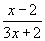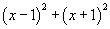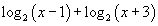index: click on a letter A B C D E F G H I J K L M N O P Q R S T U V W X Y Z A to Z index index: subject areas numbers & symbols sets, logic, proofs geometry algebra trigonometry advanced algebra & pre-calculus calculus advanced topics probability & statistics real world applications multimedia entrieswww.mathwords.com about mathwords website feedback

 Expression Any mathematical calculation or formula combining numbers and/or variables using sums, differences, products, quotients (including fractions), exponents, roots, logarithms, trig functions, parentheses, brackets, functions, or other mathematical operations. Expressions may not contain the equal sign (=) or any type of inequality. Examples:See also Equation